• 冯立伟+张成+屈福志"""摘要：针对二维对流扩散方程边值问题，采用三角形剖分，使用二维线性有限元进行计算分析。采用matlab编写了计算程序，使用算例进行了数值实验，实验结果表明数值解具有较高的计算精度。关键词...

冯立伟+张成+屈福志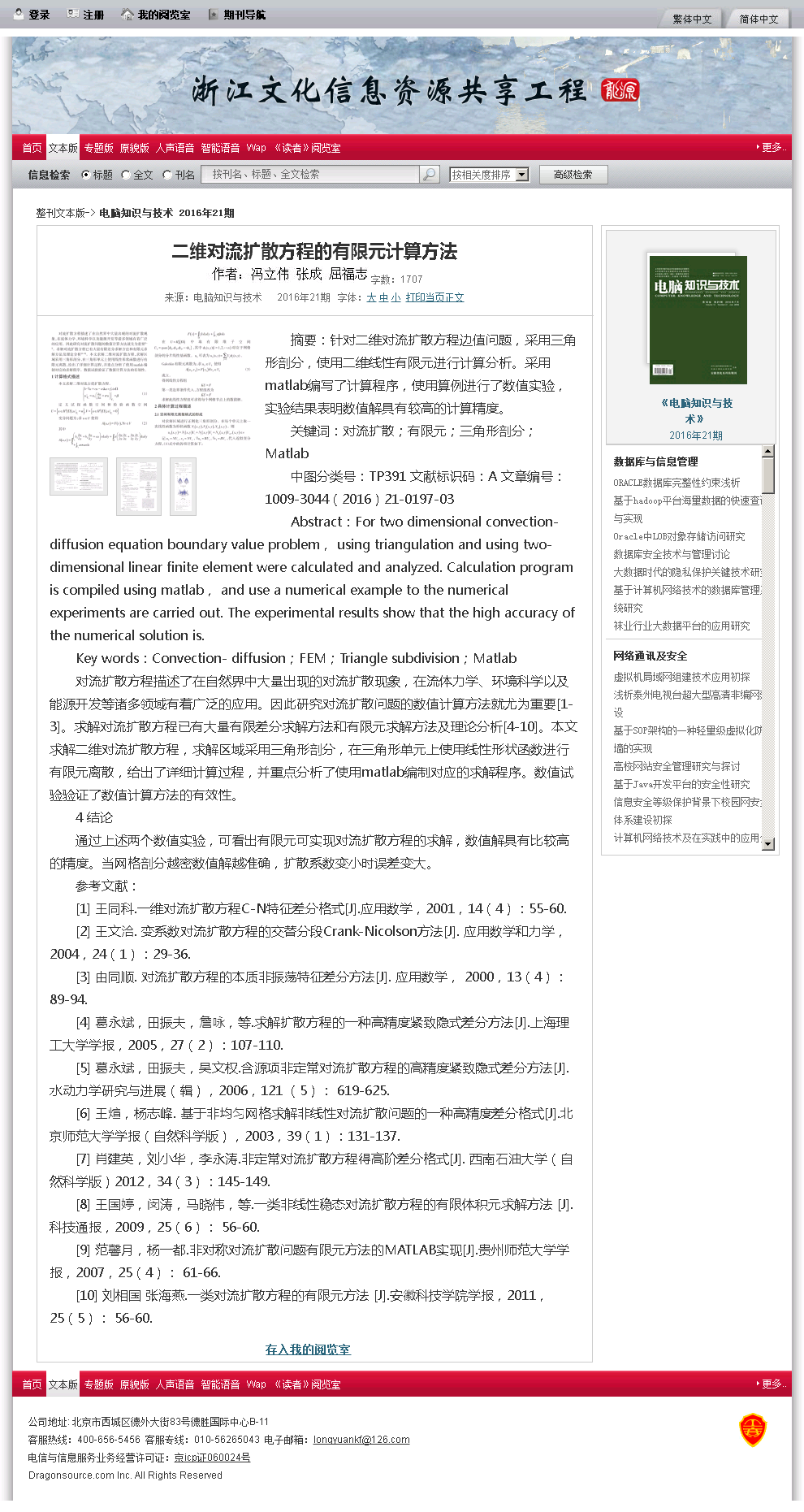"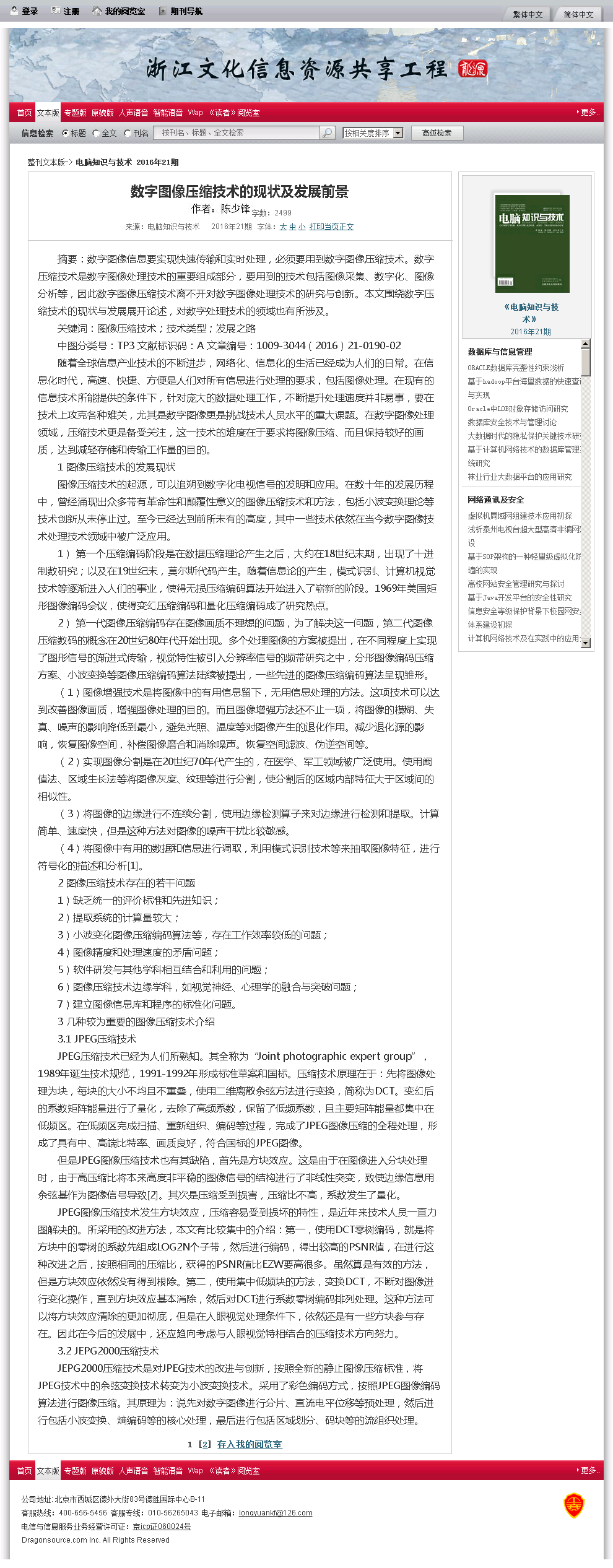"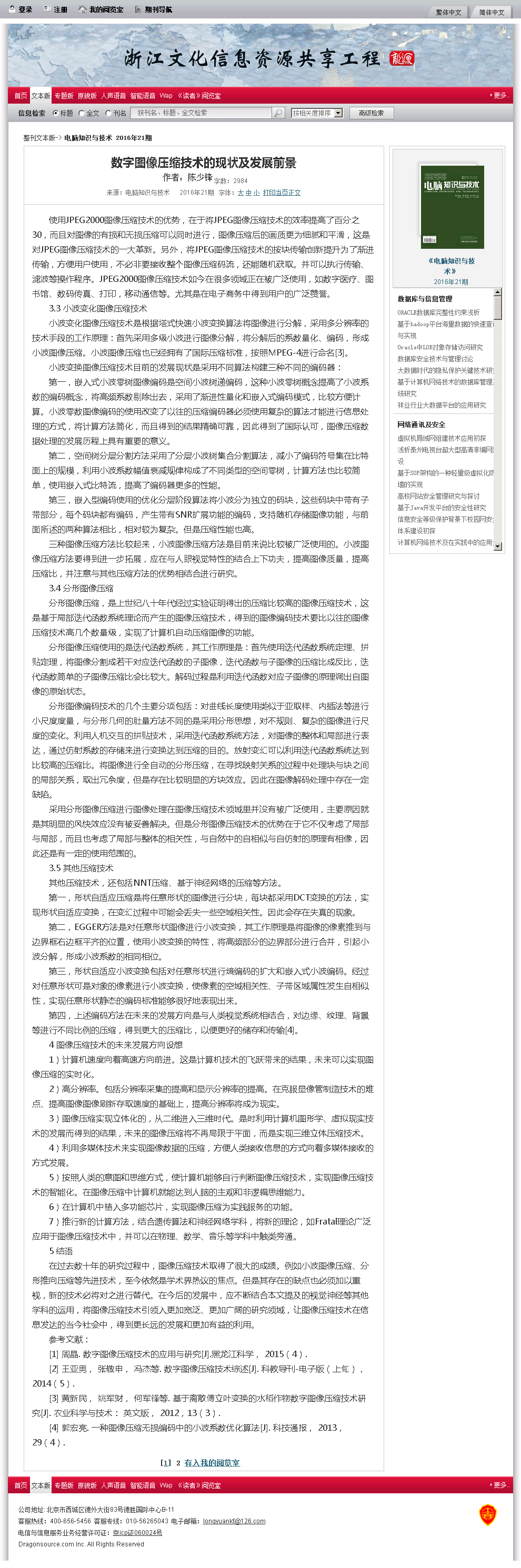"
摘要：针对二维对流扩散方程边值问题，采用三角形剖分，使用二维线性有限元进行计算分析。采用matlab编写了计算程序，使用算例进行了数值实验，实验结果表明数值解具有较高的计算精度。
关键词：对流扩散；有限元；三角形剖分；Matlab
中图分类号：TP391 文献标识码：A 文章编号：1009-3044(2016)21-0197-03
Abstract：For two dimensional convection-diffusion equation boundary value problem， using triangulation and using two-dimensional linear finite element were calculated and analyzed. Calculation program is compiled using matlab， and use a numerical example to the numerical experiments are carried out. The experimental results show that the high accuracy of the numerical solution is.
Key words：Convection- diffusion；FEM；Triangle subdivision；Matlab
对流扩散方程描述了在自然界中大量出现的对流扩散现象，在流体力学、环境科学以及能源开发等诸多领域有着广泛的应用。因此研究对流扩散问题的数值计算方法就尤为重要[1-3]。求解对流扩散方程已有大量有限差分求解方法和有限元求解方法及理论分析[4-10]。本文求解二维对流扩散方程，求解区域采用三角形剖分，在三角形单元上使用线性形状函数进行有限元离散，给出了详细计算过程，并重点分析了使用matlab编制对应的求解程序。数值试验验证了数值计算方法的有效性。
4 结论
通过上述两个数值实验，可看出有限元可实现对流扩散方程的求解，数值解具有比较高的精度。当网格剖分越密数值解越准确，扩散系数变小时误差变大。
参考文献：
 王同科.一维对流扩散方程C-N特征差分格式[J].应用数学，2001，14(4)：55-60.
 王文洽. 变系数对流扩散方程的交替分段Crank-Nicolson方法[J]. 应用数学和力学，2004，24(1)：29-36.
 由同顺. 对流扩散方程的本质非振荡特征差分方法[J]. 应用数学， 2000，13(4)：89-94.
 葛永斌，田振夫，詹咏，等.求解扩散方程的一种高精度紧致隐式差分方法[J].上海理工大学学报，2005，27(2)：107-110.
 葛永斌，田振夫，吴文权.含源项非定常对流扩散方程的高精度紧致隐式差分方法[J].水动力学研究与进展(辑)，2006，121 (5)： 619-625.
 王煊，杨志峰. 基于非均匀网格求解非线性对流扩散问题的一种高精度差分格式[J].北京师范大学学报(自然科学版)，2003，39(1)：131-137.
 肖建英，刘小华，李永涛.非定常对流扩散方程得高阶差分格式[J]. 西南石油大学(自然科学版)2012，34(3)：145-149.
 王国婷，闵涛，马晓伟，等.一类非线性稳态对流扩散方程的有限体积元求解方法 [J].科技通报，2009，25(6)： 56-60.
 范馨月，杨一都.非对称对流扩散问题有限元方法的MATLAB实现[J].贵州师范大学学报，2007，25(4)： 61-66.
 刘相国 张海燕.一类对流扩散方程的有限元方法 [J].安徽科技学院学报，2011，25(5)： 56-60.
使用JPEG2000图像压缩技术的优势，在于将JPEG图像压缩技术的效率提高了百分之30，而且对图像的有损和无损压缩可以同时进行，图像压缩后的画质更为细腻和平滑，这是对JPEG图像压缩技术的一大革新。另外，将JPEG图像压缩技术的按块传输创新提升为了渐进传输，方便用户使用，不必非要接收整个图像压缩码流，还能随机获取。并可以执行传输、滤波等操作程序。JPEG2000图像压缩技术如今在很多领域正在被广泛使用，如数字医疗、图书馆、数码传真、打印，移动通信等。尤其是在电子商务中得到用户的广泛赞誉。
3.3 小波变化图像压缩技术
小波变化图像压缩技术是根据塔式快速小波变换算法将图像进行分解，采用多分辨率的技术手段的工作原理：首先采用多级小波进行图像分解，将分解后的系数量化、编码，形成小波图像压缩。小波图像压缩也已经拥有了国际压缩标准，按照MPEG-4进行命名。
小波变换图像压缩技术目前的发展现状是采用不同算法构建三种不同的编码器：
第一，嵌入式小波零树图像编码是空间小波树递编码，这种小波零树概念提高了小波系数的编码概念，将高频系数剔除出去，采用了渐进性量化和嵌入式编码模式，比较方便计算。小波零数图像编码的使用改变了以往的压缩编码器必须使用复杂的算法才能进行信息处理的方式，将计算方法简化，而且得到的结果精确可靠，因此得到了国际认可，图像压缩数据处理的发展历程上具有重要的意义。
第二，空间树分层分割方法采用了分层小波树集合分割算法，减小了编码符号集在比特面上的规模，利用小波系数幅值衰减规律构成了不同类型的空间零树，计算方法也比较简单，使用嵌入式比特流，提高了编码器更多的性能。
第三，嵌入型编码使用的优化分层阶段算法将小波分为独立的码块，这些码块中带有子带部分，每个码块都有编码，产生带有SNR扩展功能的编码，支持随机存储图像功能，与前面所述的两种算法相比，相对较为复杂。但是压缩性能也高。
三种图像压缩方法比较起来，小波图像压缩方法是目前来说比较被广泛使用的。小波图像压缩方法要得到进一步拓展，应在与人眼视觉特性的结合上下功夫，提高图像质量，提高压缩比，并注意与其他压缩方法的优势相结合进行研究。
3.4 分形图像压缩
分形图像压缩，是上世纪八十年代经过实验证明得出的压缩比较高的图像压缩技术，这是基于局部迭代函数系统理论而产生的图像压缩技术，得到的图像编码技术要比以往的图像压缩技术高几个数量级，实现了计算机自动压缩图像的功能。
分形图像压缩使用的是迭代函数系统，其工作原理是：首先使用迭代函数系统定理、拼贴定理，将图像分割成若干对应迭代函数的子图像，迭代函数与子图像的压缩比成反比，迭代函数简单的子图像压缩比会比较大。解码过程是利用迭代函数对应子图像的原理调出自图像的原始状态。
分形图像编码技术的几个主要分项包括：对曲线长度使用类似于亚取样、内插法等进行小尺度度量，与分形几何的肚量方法不同的是采用分形思想，对不规则、复杂的图像进行尺度的变化。利用人机交互的拼贴技术，采用迭代函数系统方法，对图像的整体和局部进行表达，通过仿射系数的存储来进行变换达到压缩的目的。放射变幻可以利用迭代函数系统达到比较高的压缩比。将图像进行全自动的分形压缩，在寻找映射关系的过程中处理块与块之间的局部关系，取出冗余度，但是存在比较明显的方块效应。因此在图像解码处理中存在一定缺陷。
采用分形图像压缩进行图像处理在图像压缩技术领域里并没有被广泛使用，主要原因就是其明显的风快效应没有被妥善解决。但是分形图像压缩技术的优势在于它不仅考虑了局部与局部，而且也考虑了局部与整体的相关性，与自然中的自相似与自仿射的原理有相像，因此还是有一定的使用范围的。
3.5 其他压缩技术
其他压缩技术，还包括NNT压缩、基于神经网络的压缩等方法。
第一，形状自适应压缩是将任意形状的图像进行分块，每块都采用DCT变换的方法，实现形状自适应变换，在变幻过程中可能会丢失一些空域相关性。因此会存在失真的现象。
第二，EGGER方法是对任意形状图像进行小波变换，其工作原理是将图像的像素推到与边界框右边框平齐的位置，使用小波变换的特性，将高频部分的边界部分进行合并，引起小波分解，形成小波系数的相同相位。
第三，形状自适应小波变换包括对任意形状进行熵编码的扩大和嵌入式小波编码。经过对任意形状可是对象的像素进行小波变换，使像素的空域相关性、子带区域属性发生自相似性，实现任意形状静态的编码标准能够很好地表现出来。
第四，上述编码方法在未来的发展方向是与人类视觉系统相结合，对边缘、纹理、背景等进行不同比例的压缩，得到更大的压缩比，以便更好的储存和传输。
4 图像压缩技术的未来发展方向设想
1)计算机速度向着高速方向前进。这是计算机技术的飞跃带来的结果，未来可以实现图像压缩的实时化。
2)高分辨率。包括分辨率采集的提高和显示分辨率的提高。在克服显像管制造技术的难点、提高图像图像刷新存取速度的基础上，提高分辨率将成为现实。
3)图像压缩实现立体化的，从二维进入三维时代。是时利用计算机图形学、虚拟现实技术的发展而得到的结果，未来的图像压缩将不再局限于平面，而是实现三维立体压缩技术。
4)利用多媒体技术来实现图像数据的压缩，方便人类接收信息的方式向着多媒体接收的方式发展。
5)按照人类的意图和思维方式，使计算机能够自行判断图像压缩技术，实现图像压缩技术的智能化。在图像压缩中计算机就能达到人脑的主观和非逻辑思维能力。
6)在计算机中植入多功能芯片，实现图像压缩为实践服务的功能。
7)推行新的计算方法，结合遗传算法和神经网络学科，将新的理论，如Fratal理论广泛应用于图像压缩技术中，并可以在物理、数学、音乐等学科中触类旁通。
5 结语
在过去数十年的研究过程中，图像压缩技术取得了很大的成绩。例如小波图像压缩、分形推向压缩等先进技术，至今依然是学术界热议的焦点。但是其存在的缺点也必须加以重视，新的技术必将对之进行替代。在今后的发展中，应不断结合本文提及的视觉神经等其他学科的运用，将图像压缩技术引领入更加宽泛、更加广阔的研究领域，让图像压缩技术在信息发达的当今社会中，得到更长远的发展和更加有益的利用。
参考文献：
 周晶. 数字图像压缩技术的应用与研究[J].黑龙江科学， 2015(4).
 王亚男， 张敬申， 冯杰等. 数字图像压缩技术综述[J]. 科教导刊-电子版(上旬)， 2014(5).
 黄新民， 姚军财， 何军锋等. 基于离散傅立叶变换的水稻作物数字图像压缩技术研究[J]. 农业科学与技术： 英文版， 2012，13(3).
 郭宏亮. 一种图像压缩无损编码中的小波系数优化算法[J]. 科技通报， 2013，29(4).

展开全文• 总结了近些年出现的针对二维对流扩散方程给出的多种差分格式；随后对一维模型给出了一种基本二阶格式，然后将结果直接推广应用到二维情形，得到一种新的无条件稳定的二阶五点差分格式；最后通过数值实验与前面诸多...
• 由 Sreetam Bhaduri 和 Shekhar Mishra 开发。
• “ UNSTEADY_CONVECTION_DIFFUSION”脚本用双线性四边形元素求解对流扩散问题的二标量方程。 空间离散化是通过标准的Galerkin方法执行的。 对于时间积分，已经实施了 theta 方法。 根据 theta 的值，获得这些方案...
• React扩散方程式基于Lengyel-Epstein模型的模式机器学习（20200115〜20200730） 在科学计算实验室中，这是我的第个机器学习项目。 我们的目的是针对使用机器学习和特征工程技术从图灵模型生成的图案图像进行...
• 有限体积法 求解 一维维对流扩散问题 ，一维稳态问题，采用中心差分并与解析解比较。
• 该程序采用有限差分方法（隐式和显式）仿真了一维和二维域扩散方程。该程序采用有限差分方法（隐式和显式）仿真了一维和二维域扩散方程。该程序采用有限差分方法（隐式和显式）仿真了一维和二维域扩散方程
• 一维线性平流方程使用五种有限差分方案（全部显式）来求解。 First Order Upwind、Lax-Friedrichs、Lax-Wendroff、Adams Average (Lax-Friedrichs) 和 Adams Average (Lax-Wendroff)。 使用启发式时间步长。 使用...
• 种时间分数阶对流扩散方程的隐式差分近似，马亮亮，田富鹏，考虑时间分数阶对流扩散方程,将一阶的时间导数用分数阶导数 替换,给出了种计算有效的隐式差分格式,并证明了这个隐式差分格式是�
• 个二对流扩散问题，对流在X方向， 扩散作用在Y 方向，所以方程为 dC/dt=D*d2C/dy2-V*dC/dx (注：D= Diffusion coefficient, C=concentration, V=流体速度，并假设它与表面的垂直方向Y成正比，即：V=a*Y....

是一个二维的对流扩散问题，对流在X方向， 扩散作用在Y 方向，所以方程为 dC/dt=D*d2C/dy2-V*dC/dx (注：
D= Diffusion coefficient, C=concentration, V=
流体速度，并假设它与表面的垂直方向Y成正比，即：V=a*Y. d2C/dy2表示浓度对Y的二阶导数。 ) 边界条件为：
1 ,C = C0 when y = 0 and 0
(表示在表面(y =
0)存在药品(扩散物质)的区域(0
2, C = 0 when y> 0.1cm for all x
(表示对难容物质来说，由于流动的因素，离表面一定距离后不存在溶质)
3, C = 0 when x = 0 for all y (因为在存在药品区域之前是 没有浓度的)
4, C = 0 when y = 0 and x> L (表示
在不存在药品的表面也没有浓度)
本人用论文中介绍的显示差分算法在MATLAB 编程 [C(i , j , k+1)- C(i , j , k)]/dt =
D/(dy)^2 * [ C(i , j-1 , k) - 2*C(i , j , k) + C(i , j+1 , k)] -
V/dx * [C(i , j , k) - C(i -1, j , k)]
下面是我的代码：
问题是，虽然可以得到仿真(步长设置不好还容易发散)，但是明显没有对流项的作用，画出的图应该是从左到右被“冲”的感觉，实在找不到问题出在哪儿了，恳请大侠赐教，不胜感谢！
%%%%%%%%%%%%%%%%%%
clear
clc
D = [0 ,2 , 0 , 0.1 ] %%%% region of
0
0
Mx =200
My =100
N = 1000
T = 10
dif = 9.86*10^(-6) %%%%diffusion coefficient
alfa = 4.25
visco = 0.89
dx = (D(2) - D(1))/Mx;
dy = (D(4) - D(3))/My;
dt = T/N; t = [0:N]*dt;
%initialization and boundary condition
for j = 1:Mx + 1
for i = 1:My + 1
u(i,j) = 0 ;
end
end
for j = 1:Mx /2
u(1,j) = 1;
u(My+1,j) = 0;
end
ry = dif*dt/(dy*dy);
for k = 1:N
u_1 = u; t = k*dt;
for i= 2:My+1
vx = alfa*i*D(4)/My; %%%%to be
rx = vx*dt/dx;
rx1 = 1 - rx;
for j =2 : Mx
u(i , j )=ry*(u_1(i,j-1)-2*u_1(i , j)+u_1(i , j+1)) +rx1*u_1(i ,
j)+rx*u_1(i-1 , j);
end
end
for i = 1: My + 1
u(i , Mx + 1)=u(i , Mx);
end
end
i = 1:Mx+1;
j= 1: My+1;
[I, J]=meshgrid(i,j);
surf(I , J , u)
figure;
mesh(I, J , u)

展开全文• 求解一维对流方程的代码，使用内含一维迎风格式、二维迎风格式等数值格式求解。初始场可设置三角波和阶梯波，输出dat格式文件可以用tecplot或matlab查看。
• 有限体积法 求解 一维维对流扩散问题 ，一维稳态问题，采用中心差分并与解析解比较。一维稳态 乘方格式
• 初次接触方程的离散与求解，问题虽多，但也是探索新事物的起点。


小乌龟学河流数值模拟
一维纯对流方程的求解过程问题描述解析解数值解迎风格式求解特征线法求解
算例工况与计算结果敏感性分析浓度分布均匀性的影响（不算敏感性，单纯考虑对数值求解精度的影响）库朗数影响

实践中的认知与遇到的错误迎风格式的理解特征线法离散过程分析

Δ

x

\Delta{x}

与

d

x

dx

的错误理解导致对特征线法的认知错误错误认知内容错误认知产生的原因错误认知产生的结果图片与MATLAB代码
Python代码1（失败）遇到报错信息1：TypeError with ufunc bitwise_xor遇到的报错信息2：TypeError: only length-1 arrays can be converted to Python scalars
Python代码2Python代码3遇到的问题一：波形错位
Python代码4MATLAB代码(成功)Matlab报错信息1:矩阵求幂的维度不正确

一维纯对流方程的求解过程
问题描述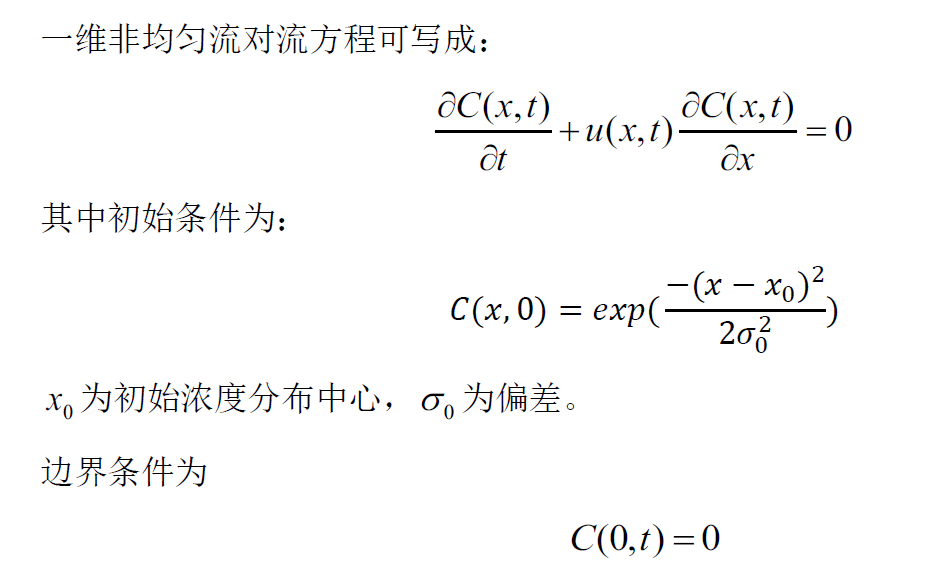解析解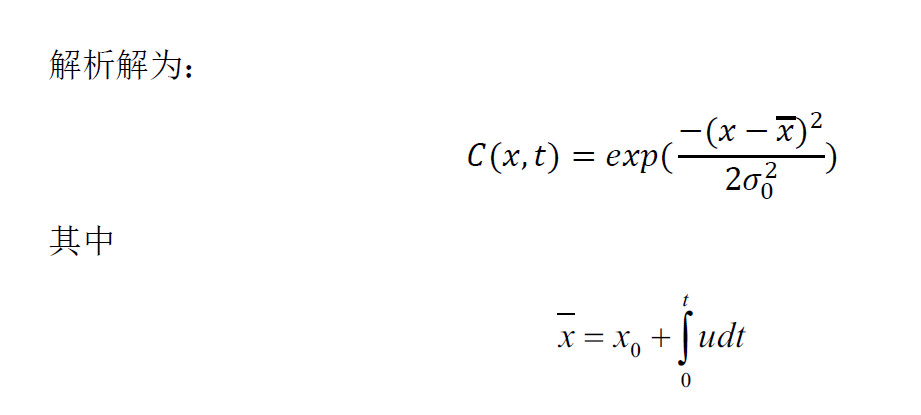数值解
迎风格式求解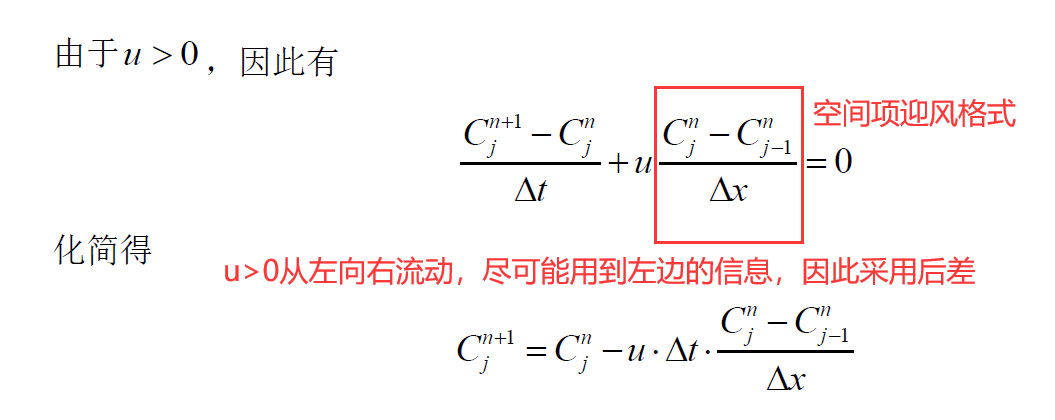特征线法求解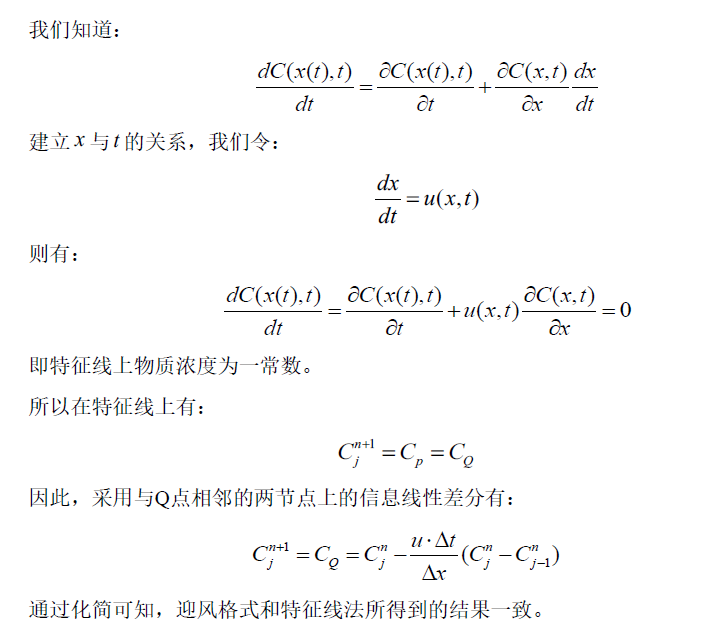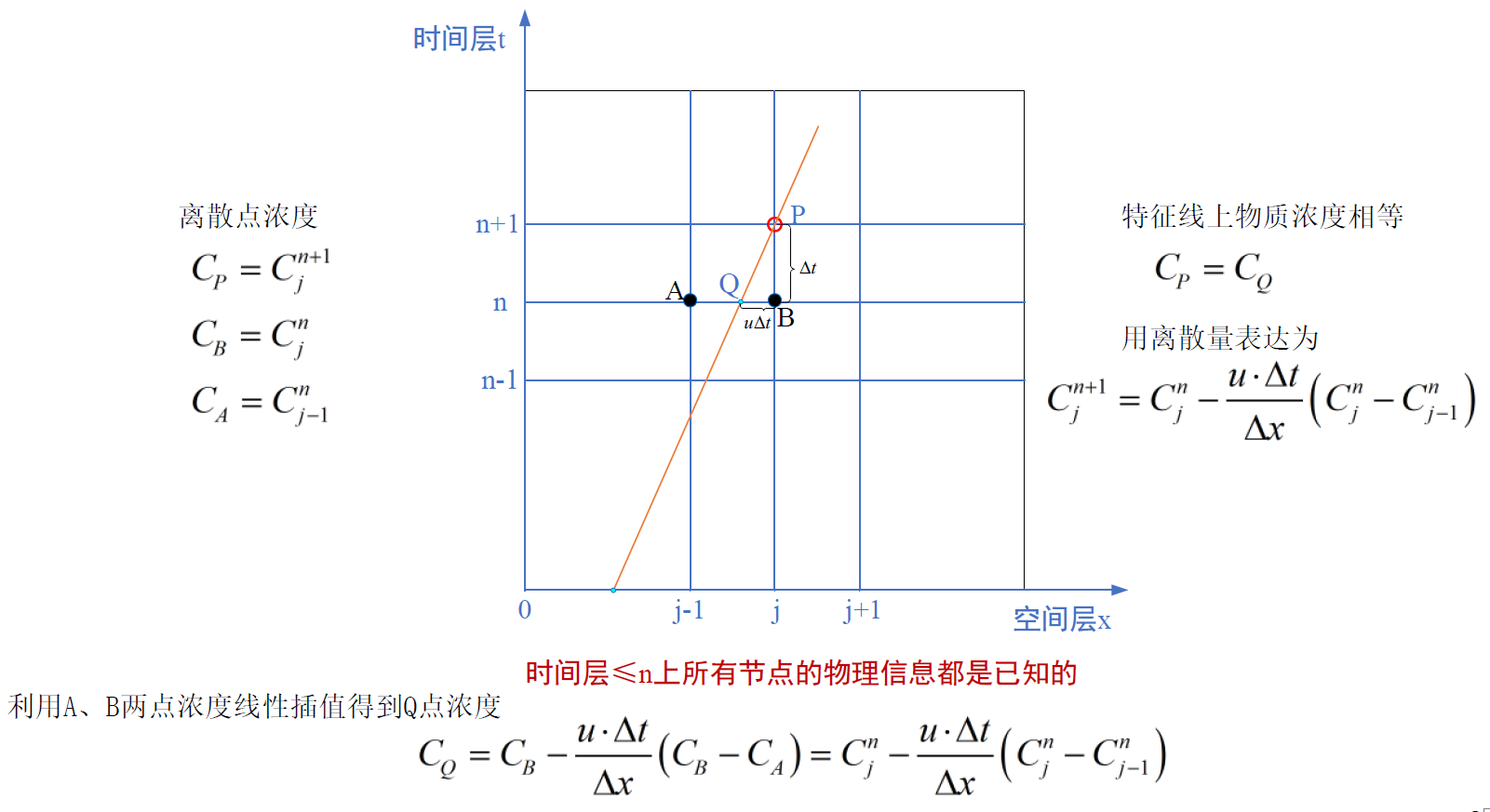算例工况与计算结果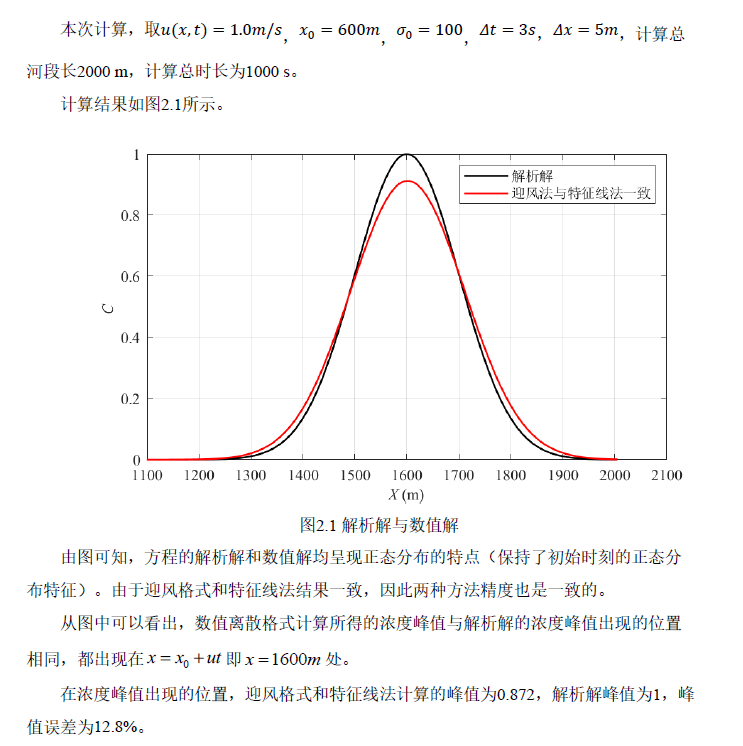% Matlab代码
% This is a  First Order Upwind for Advection Equation
clear
clc

% Set the parameters
xmin = 0;
xmax = 2000;
N = 400;
dx = (xmax-xmin)/N;
tmin = 0;
tmax = 1000;
dt = 3;
u = 1;
sigma = 100;
x0 = 600;

% Set the initial condition
x = linspace(xmin, xmax+dx, N+1);
C0 = exp( -(x-x0).^2/sigma^2*0.5);
C0(1) = 0; %MATLAB Array index start from 1

Cplus = C0;
nstep = tmax/dt;

for n = 1:nstep

Ctemp  = Cplus;
% calculate the new value
for i = 2:N+1
Cplus(i) = Ctemp(i) - u*dt*(Ctemp(i) - Ctemp(i-1))/dx;
end

end

% calculate exact solution
Cexact = exp( -(x-x0-u*tmax).^2/sigma^2*0.5);

% visualization
figure(1)
L = plot(x,Cexact,'k-',x,Cplus,'r-','linewidth',1.2);
legend(L,'解析解','\sigma0=110','location','NorthEast','FontName','宋体');
xlabel('\itX \rm(m)');
ylabel('\itC \rm');
axis([1100 2100 0 1]);
set(gca,'FontName','Times New Roman','Fontsize',10.5);
set(get(gca,'xlabel'),'FontName','Times New Roman','Fontsize',10.5);
set(get(gca,'ylabel'),'FontName','Times New Roman','Fontsize',10.5);
grid on;
set(gcf,'PaperUnits','centimeter','PaperPosition',[0 0 16 9]);

敏感性分析
浓度分布均匀性的影响（不算敏感性，单纯考虑对数值求解精度的影响）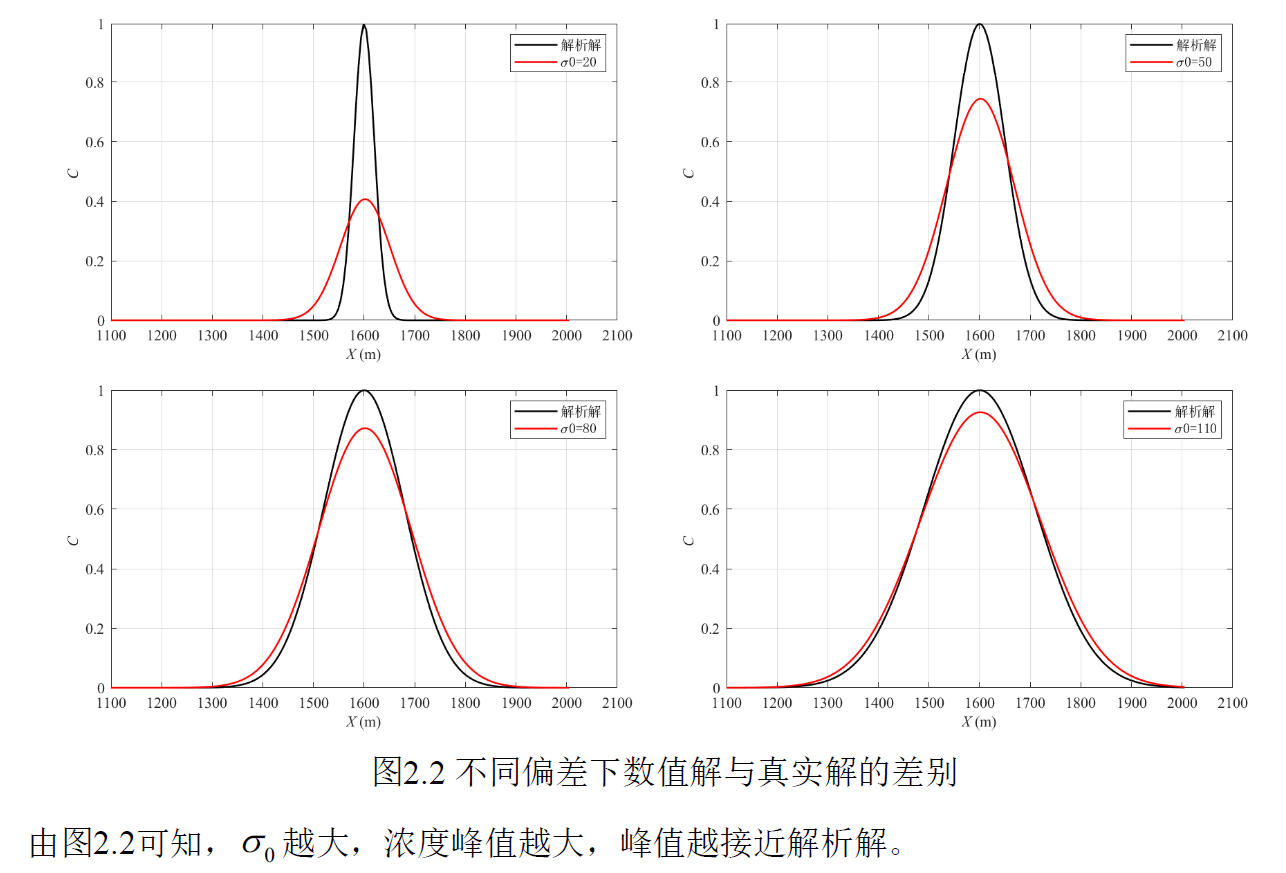说明不同工况的计算精度是不同的。
库朗数影响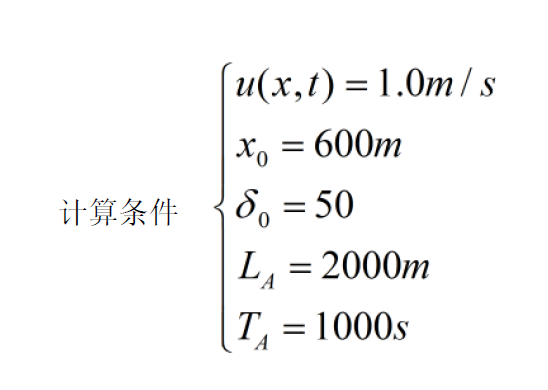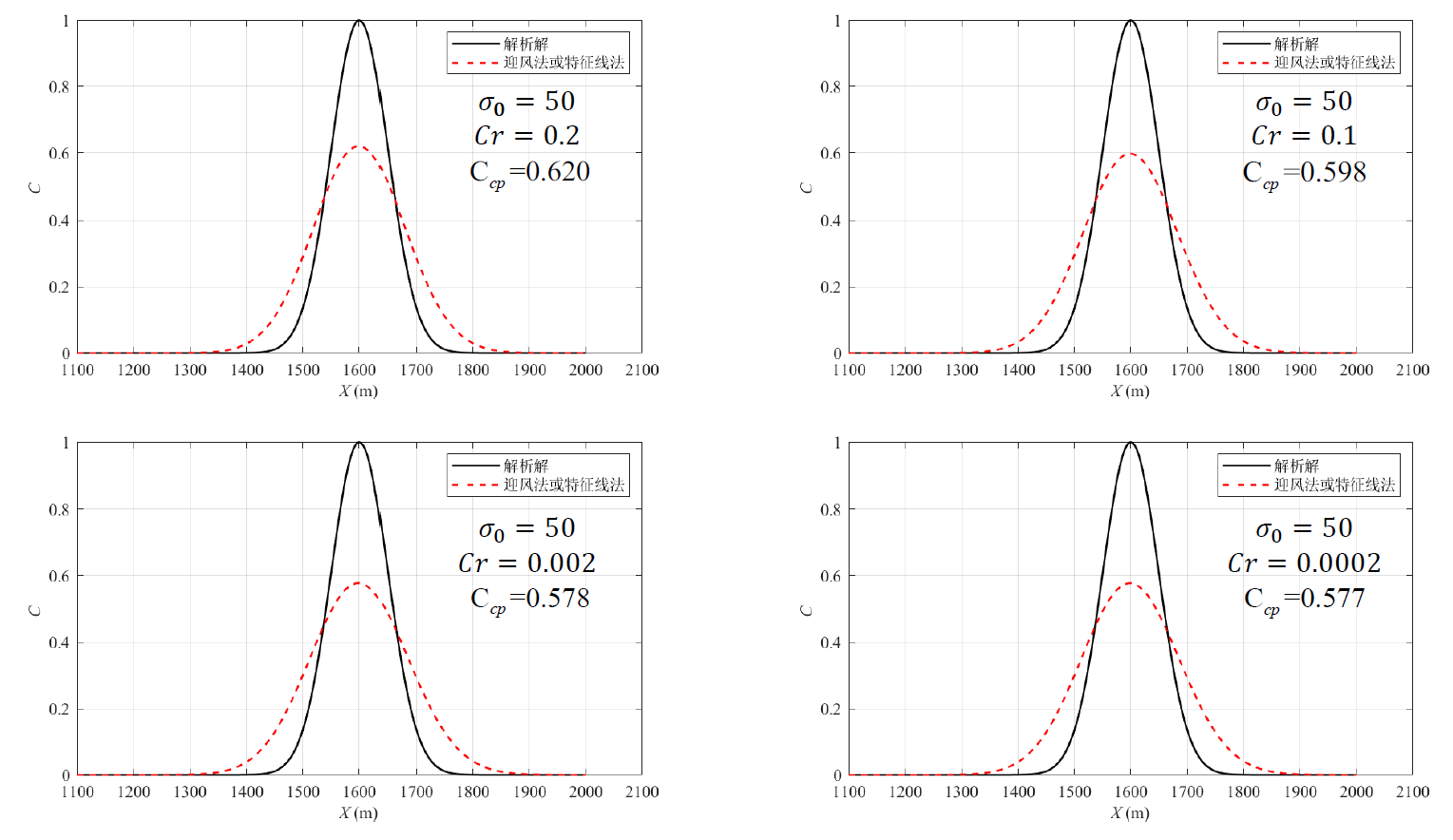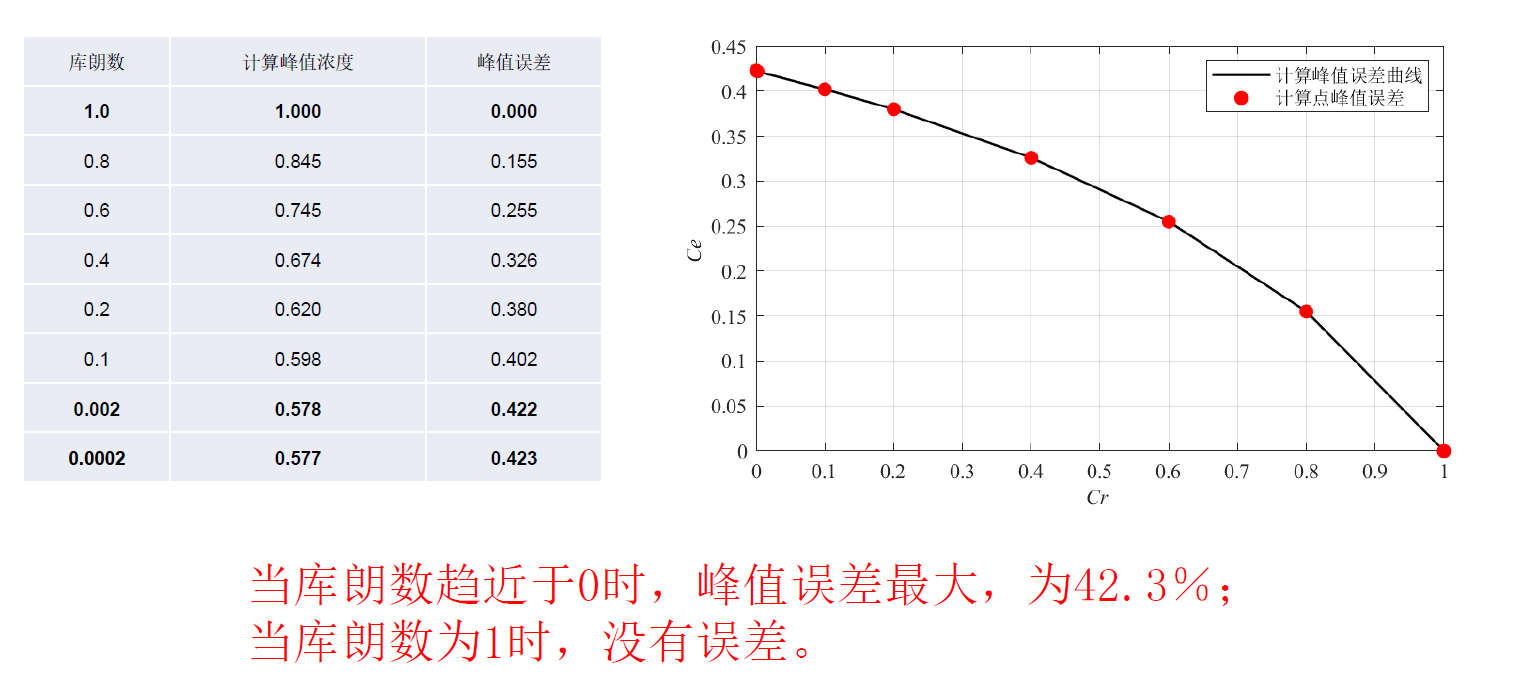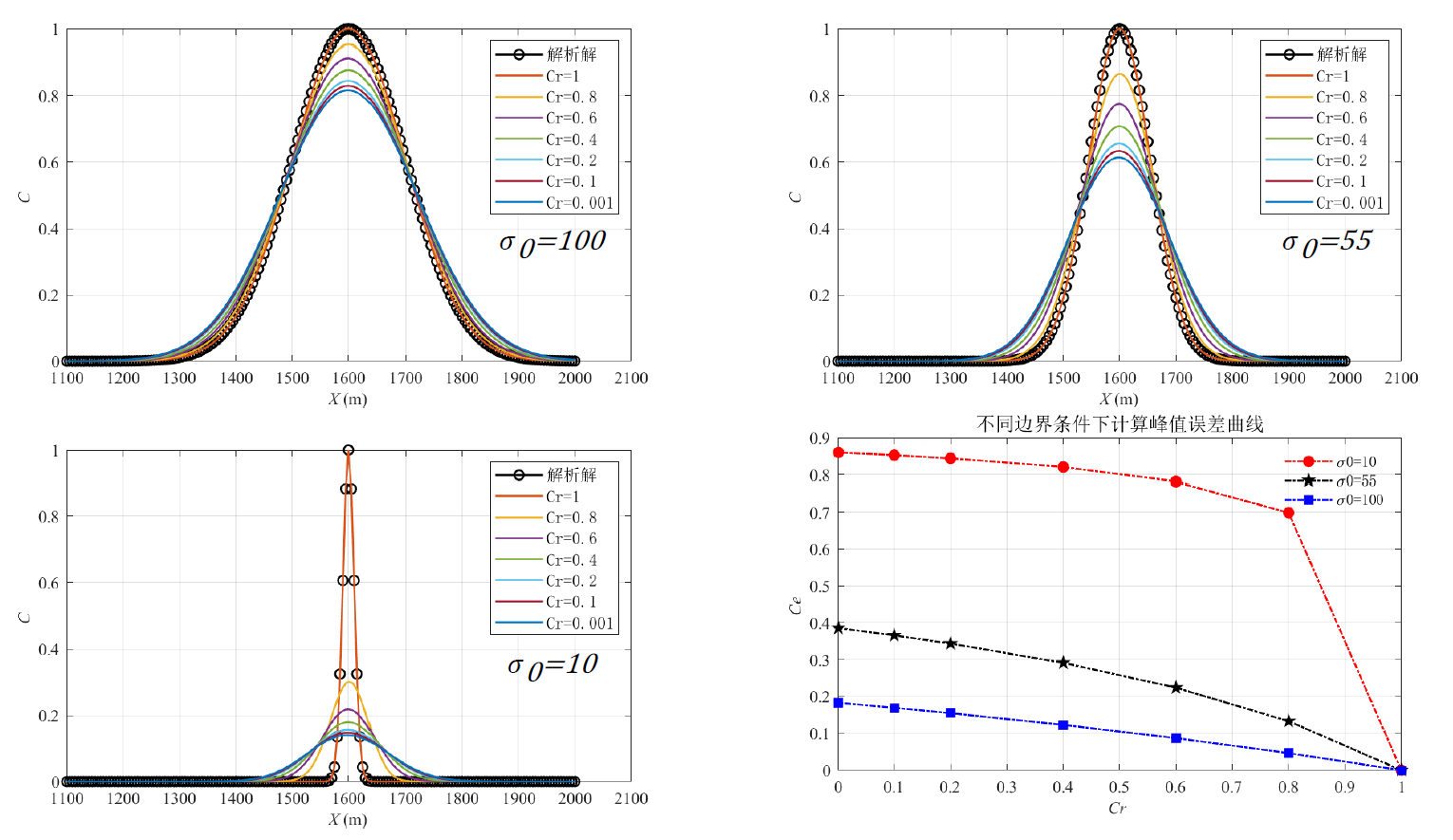实践中的认知与遇到的错误
迎风格式的理解
文章链接 对流项具有方向性，在对流项主导的运动里，某个点受到上游的影响远大于下游。迎风格式就是逆着对流的方向进行差分，以利用到更多的上游信息。假设从左到右是正方向：那么如果对流从左到右，迎风格式就是构建后差；如果对流从右到左，就是构建前差。正因为风就是一种强对流的运动，迎风格式就是逆着风吹来的方向构建差分格式，这个名字取得很形象。
特征线法离散过程分析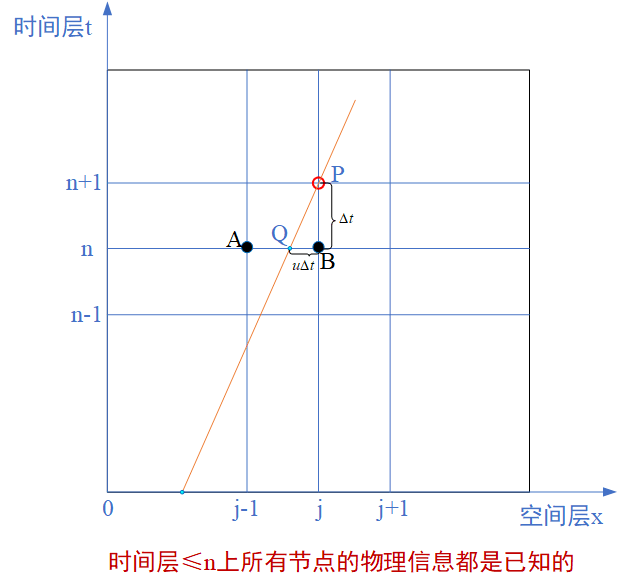方程中所有的物理变量：本一维纯对流方程中物理变量有三个，即物质浓度

C

C

，时间

t

t

，空间

x

x

。 全知物理量信息：

A

:

(

x

=

x

j

−

1

,

t

=

t

n

,

C

=

C

j

−

1

n

)

A:(x=x_{j-1},t=t_n,C=C_{j-1}^{n})

B

:

(

x

=

x

j

,

t

=

t

n

,

C

=

C

j

n

)

B:(x=x_{j},t=t_n,C=C_{j}^{n})

半知物理量信息（红色表示未知，黑色表示已知）：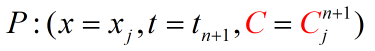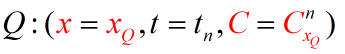Q点的物质浓度C和空间位置x都是未知的。但是，我们知道时空特征线关系式，我们还知道与Q处于同一时间层的两相邻节点A、B的所有信息。因此： 利用特征线关系可以求得Q点相对于A或B的空间位置； 然后对A、B两点采用线性插值得到Q点的物质浓度； 根据特征线上任意一点的物质浓度相等可得到待求量P点的物质浓度。

Δ

x

\Delta{x}

与

d

x

dx

的错误理解导致对特征线法的认知错误
本案例采用特征线法和迎风法分别离散上述一维纯对流方程，得到的离散方程都相同，即：

C

j

n

+

1

=

C

j

n

−

u

Δ

t

Δ

x

(

C

j

n

−

C

j

−

1

n

)

C_{j}^{n+1}=C_{j}^{n}-\frac{u\Delta{t}}{\Delta{x}}(C_{j}^{n}-C_{j-1}^{n})

错误认知内容

以下内容是2021年5月7日的错误认识： 最初，由于两者的离散方程相同，所以我错误地认为特征线法与迎风法求得的数值解是相同的。特征线法的前提条件是认为

d

x

d

t

=

u

\frac{dx}{dt}=u

，所以特征线法的离散方程一定要满足这个条件，即时间和空间的离散是受到限制的。所以，本例一维纯对流方程采用特征线时离散的方程应该为：

C

j

n

+

1

=

C

j

−

1

n

C_{j}^{n+1}=C_{j-1}^{n}

也就是迎风法离散方程后库朗数取1时的离散方程：

C

r

=

u

Δ

t

Δ

x

=

1

Cr=\frac{u\Delta{t}}{\Delta{x}}=1

错误认知产生的原因
错误地认为

Δ

x

\Delta{x}

与

d

x

dx

是相等的，即认为两者表示的含义相同。实际上，两者有着本质区别：

Δ

x

=

x

j

−

x

j

−

1

\Delta{x}=x_{j}-x_{j-1}

是两个空间点之间的距离，

Δ

x

\Delta{x}

的取值可以很大，也可以趋近于0。当

Δ

x

\Delta{x}

趋近于0时与

d

x

dx

等价。而

d

x

dx

表示

x

x

的微小增量，微小可以理解为趋近于0。所以，在空间步长

Δ

x

\Delta{x}

取值的任意性下，

d

x

d

t

=

u

\frac{dx}{dt}=u

不能推理出

Δ

x

Δ

t

=

u

\frac{\Delta{x}}{\Delta{t}}=u

。对于本案例采用特征线法的离散过程，我们可以这样理解：

Δ

t

\Delta{t}

是一个微小量（即使其取值较大，我们依旧可以假定其为相对微小量），则

Δ

t

=

d

t

\Delta{t}=dt

，所以

d

x

=

u

Δ

t

dx=u\Delta{t}

（

d

x

≤

Δ

x

dx≤\Delta{x}

）,所以

C

j

n

+

1

有

很

大

的

可

能

性

与

C

j

−

1

n

C_{j}^{n+1}有很大的可能性与C_{j-1}^{n}

不相等。
错误认知产生的结果图片与MATLAB代码
2021年5月7日更新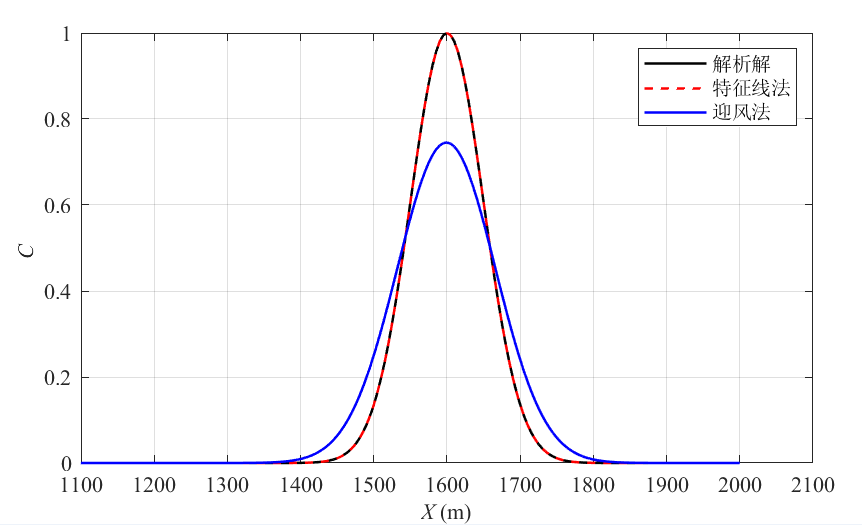% 再谈特征线法与迎风法
clear
clc

% Set the parameters
xmin = 0;
xmax = 2000;
N = 400;
dx = (xmax-xmin)/N;
tmin = 0;
tmax = 1000;
dt = 3;%换算成迎风时应该为5，迎风法总的时间步长将用5来换算
u = 1;
sigma = 50;
x0 = 600;
nstep = tmax/dt;

% Set the initial condition
x = linspace(xmin, xmax, N+1);
C0 = exp( -(x-x0).^2/sigma^2*0.5);
C0(1) = 0; %MATLAB Array index start from 1

Ctz = C0;%给特征线法的变量赋初值
Cyf = C0;%给迎风法的变量赋初值

% 特征线法求解
for n = 1:200 %nstep按dt=5换算过来的

Ctemp  = Ctz;
% calculate the new value
for i = 2:N+1
Ctz(i) = Ctemp(i-1);
end

end

% calculate exact solution
Cexact = exp( -(x-x0-u*tmax).^2/sigma^2*0.5);

% 迎风法求解
for n = 1:nstep

Ctemp  = Cyf;
% calculate the new value
for i = 2:N+1
Cyf(i) = Ctemp(i) - u*dt*(Ctemp(i) - Ctemp(i-1))/dx;

end

end

% visualization
L = plot(x,Cexact,'k-',x,Ctz,'r--',x,Cyf,'b-','linewidth',1.2);
legend(L,'解析解','特征线法','迎风法','location','NorthEast','FontName','宋体');
xlabel('\itX \rm(m)');
ylabel('\itC \rm');
axis([1100 2100 0 1]);
set(gca,'FontName','Times New Roman','Fontsize',10.5);
set(get(gca,'xlabel'),'FontName','Times New Roman','Fontsize',10.5);
set(get(gca,'ylabel'),'FontName','Times New Roman','Fontsize',10.5);
grid on;
set(gcf,'PaperUnits','centimeter','PaperPosition',[0 0 16 9]);

离散表达式后续补充……
Python代码1（失败）
import numpy as np
import math
import matplotlib.pyplot as plt

def LinearConv(nx):
x = np.linspace(0,6,nx)
Lx = 6 #x为x方向的总长度
dx = Lx/(nx-1) #dx为空间网格步长，nx为节点数
nt = 20 #总的时间步长
Courant = 0.8 #Courant数
u = 1 #假设对流为常数
dt = Courant*dx/u #时间步长

#未知参数设定
x_0 = 3 #初始浓度分布中心
sigma_0 = 1 #偏差

#初始条件和边界条件
C[0:len(nx)] = math.exp(-(x - x_0)**2/(sigma_0)**2*0.5) #初始条件C(x,0)的表达式
C = 0
#将初始图像画出来
plt.plot(x,C,'r',linewidth=3,label='init')

#计算20个时间步后的图像
Cn = math.exp((x - x_0)^2/(sigma_0)^2*(-0.5))
for n in range(nt):
Cn = C.copy() #后面的计算会改变C，所以将C拷贝到Cn
for i in range(1,nx):
C[i] = Cn[i] - u * dt / dx *(Cn[i] - Cn[i-1])

plt.plot(x,u,'b',linewidth = 3,label = 'current')
plt.xlabel('distance')
plt.ylabel('concentration')
plt.legend()
plt.show()

LinearConv(601)

遇到报错信息1：TypeError with ufunc bitwise_xor
具体报错信息：TypeError: ufunc ‘bitwise_xor’ not supported for the input types,and the inputs could not be safely coerced to any supported types according to the casting rule ‘‘safe’’ .
解决办法：Python中平方的表示不能用【∧

2

2

】，正确的语法是【

∗

∗

2

**2

】。 TypeError with ufunc bitwise_xor解决办法来源
遇到的报错信息2：TypeError: only length-1 arrays can be converted to Python scalars

暂未找到解决办法

Python代码2
遇到的报错信息2暂时无法解决，通过联想到XY曲线绘制时的一一对应关系，退而求其次初步成功。 Python绘制指数函数（解决办法来源）
import numpy as np
import math
import matplotlib.pyplot as plt

X = np.linspace(0,6,61)
x_0 = 3
sigma_0 = 1
dx = 0.1
nt = 20
u = 0.5
dt = 0.08

def phi_func(x):
phi = math.exp(-(x - x_0)**2/(sigma_0)**2*0.5)
return phi

Phi = [phi_func(x) for x in X] #因为解决不了数组元素一一对应的问题，才出此下策
C = Phi
C = 0
plt.plot(X,Phi,'r',linewidth=3,label='init')

Cn = Phi
for n in range(nt):
Cn = C #list没有copy
for i in range(1,61):
C[i] = Cn[i]-u*dt/dx*(Cn[i]-Cn[i-1])

plt.plot(X,C,'b',linewidth=3,label='current')
plt.xlabel('distance')
plt.ylabel('concetration')
plt.legend()
plt.show()

效果图如下：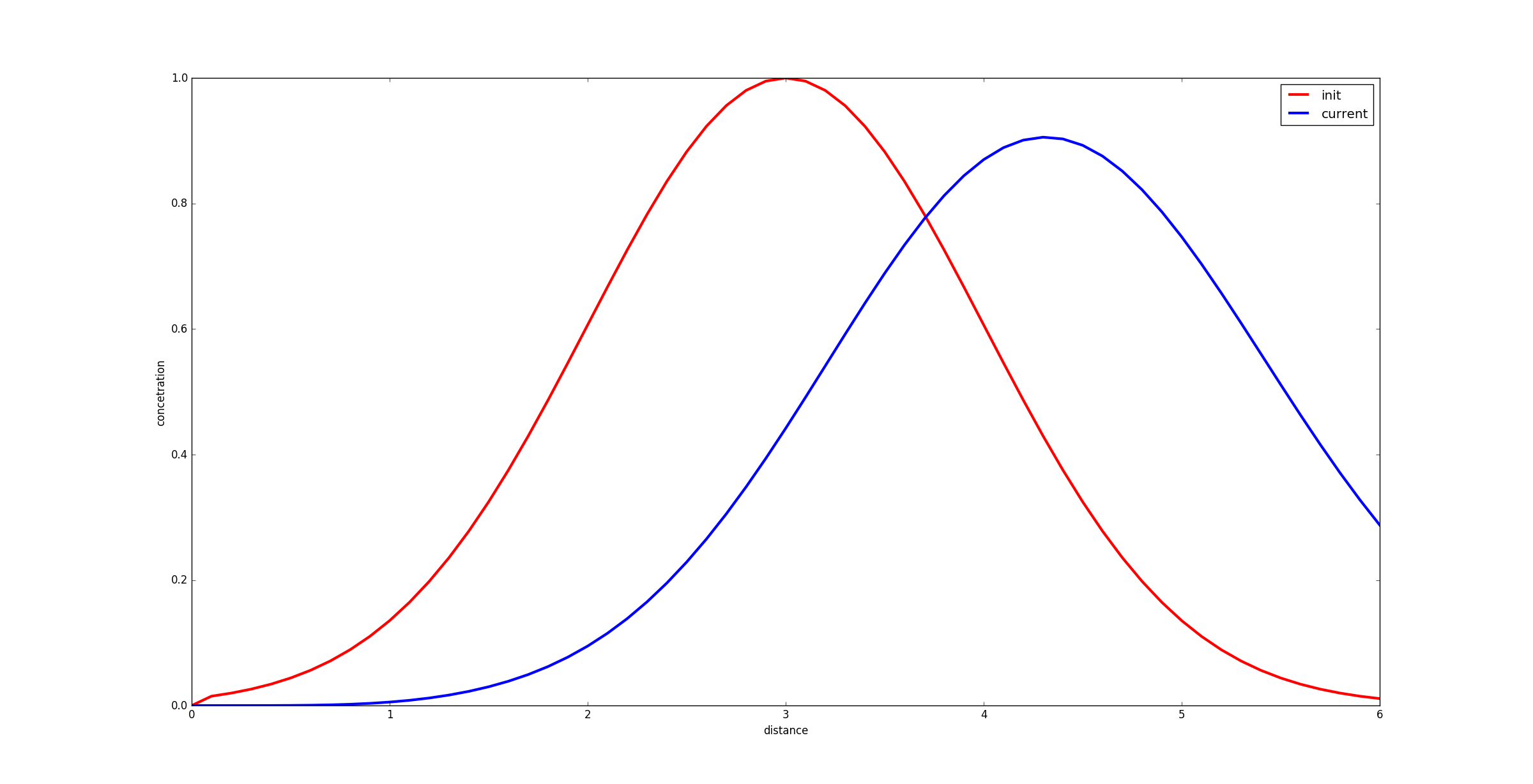起初对解析曲线的绘制存在误解，理解成对空间和时间都要离散。突然才发现，数值计算得到的就是给定时间的最后结果，因此解析解也仅需要最后时刻即可，即时间在解析函数中是一个固定值。不过，把解析函数随时间变化的动态图做出来也是值得考虑的。 Python绘制函数曲线（绘制解析解时的解决办法来源）
Python代码3
import numpy as np
import math
import matplotlib.pyplot as plt
X = np.linspace(0,2000,401)
x_0 = 500
sigma_0 = 100
dx = 5
nt = 401#总的时间步长
u = 0.3
dt = 5

def phi_func(x):
phi = math.exp(-(x - x_0)**2/(sigma_0)**2*0.5)
return phi

Phi = [phi_func(x) for x in X] #因为解决不了数组元素一一对应的问题，才出此下策
C = Phi
C = 0
plt.plot(X,C,'r',linewidth=3,label='init')

#Cn = Phi
for n in range(nt):
Cn = C #list没有copy属性，所以直接整个赋值即可
for i in range(1,401):
C[i] = Cn[i]-u*dt/dx*(Cn[i]-Cn[i-1])

plt.plot(X,C,'b',linewidth=3,label='current')
#怎么对受x和t影响的C进行显示呢？
C = []
for x in X:
Cn = math.exp(-(x - x_0-u*(nt-1)*dt)**2/(sigma_0)**2*0.5)
C.append(Cn)

plt.plot(X,C,'k',linewidth=3,label='theory resolution ')

plt.xlabel('distance')
plt.ylabel('concetration')
#plt.xlim(0, 2000)

plt.legend()
plt.show()

遇到的问题一：波形错位
蓝色波形应该与黑色波形相位一致，但是两者却是错开的。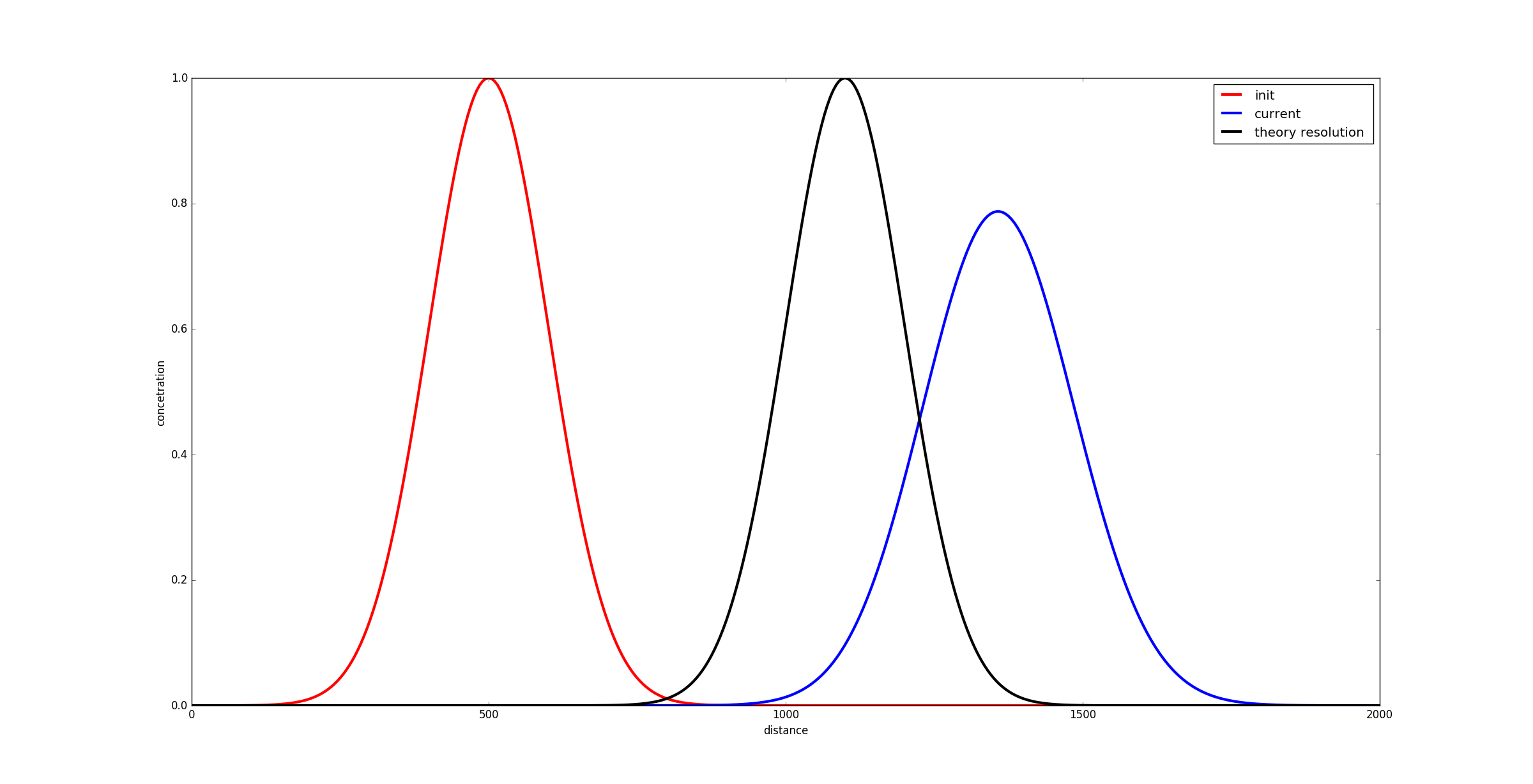Python代码4
为什么迭代步与预计的时间步有一定关系偏差才能得到原本正确的解，此处的关系偏差已经不能从物理意义上去理解。 Python中
import numpy as np
import math
import matplotlib.pyplot as plt

X = np.linspace(0,2000,401)
x_0 = 500
sigma_0 = 150
dx = 5
nt = 601#总的时间步长
u = 0.3
dt = 5

def phi_func(x):
phi = math.exp(-(x - x_0)**2/(sigma_0)**2*0.5)
return phi

Phi = [phi_func(x) for x in X] #因为解决不了数组元素一一对应的问题，才出此下策
C = Phi
C = 0
plt.plot(X,C,'r',linewidth=3,label='init')

#Cn = Phi
steps = int(nt-(nt-1)*u)#range()函数内部只能是整型，u是float所以导致整个表达式为float，因此强制转换类型
for n in range(steps):#为什么迭代步为nt-(nt-1)*u才对，如nt=201,则迭代步为141

Cn = C #list没有copy属性，所以直接整个赋值即可

for i in range(1,401):
C[i] = Cn[i]-u*dt/dx*(Cn[i]-Cn[i-1])

plt.plot(X,C,'b',linewidth=3,label='current')
#怎么对受x和t影响的C进行显示呢？
C = []
for x in X:
Cn = math.exp(-(x - x_0-u*(nt-1)*dt)**2/(sigma_0)**2*0.5)
C.append(Cn)

plt.plot(X,C,'k',linewidth=3,label='theory resolution ')

plt.xlabel('distance')
plt.ylabel('concetration')
#plt.xlim(0, 2000)

plt.legend()
plt.show() #显示出图像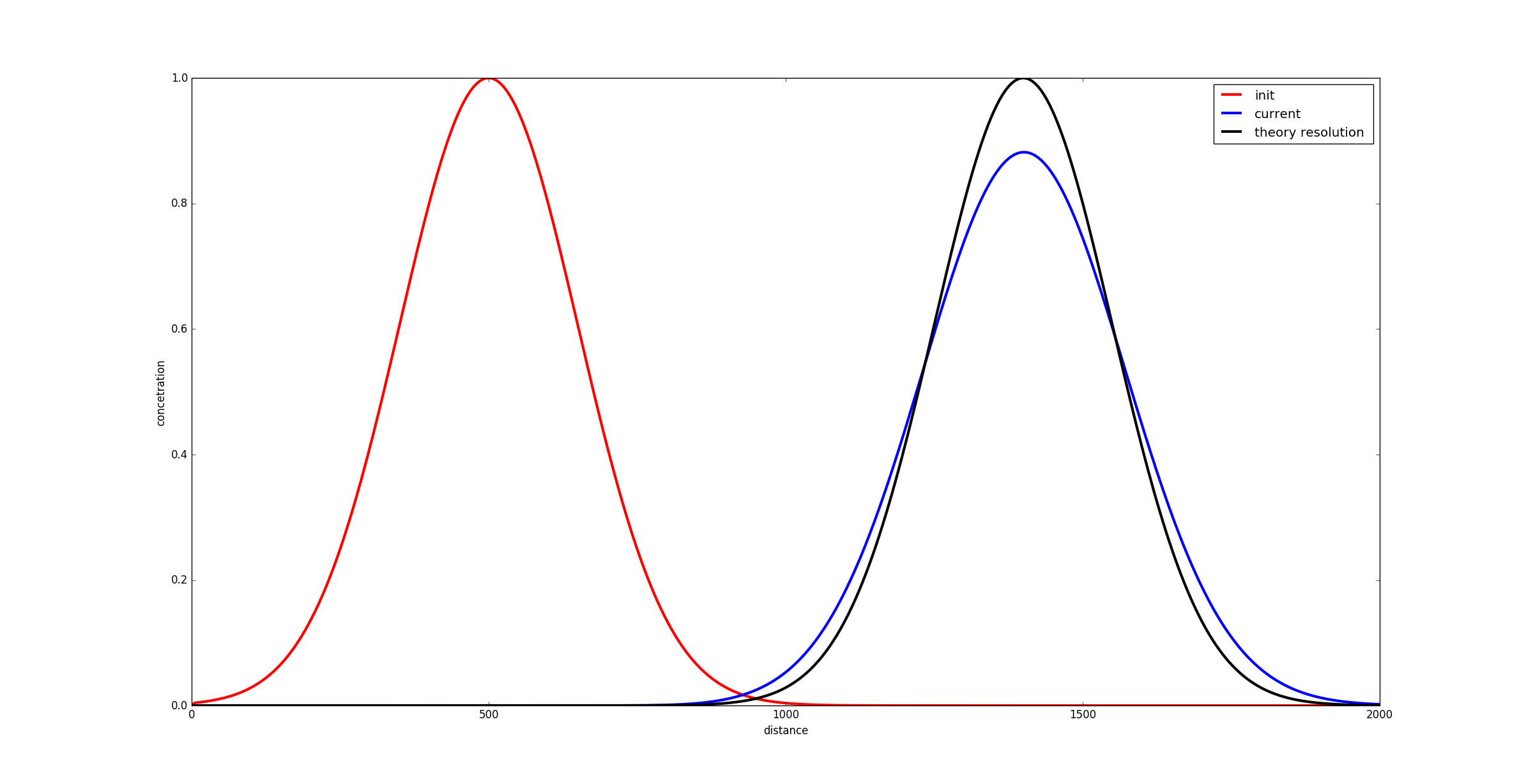MATLAB代码(成功)
MATLAB帮助来源
% This is a demo of First Order Upwind for Advection Equation
clear
clc

% Set the parameters
xmin       = 0;
xmax       = 2000;
N          = 400;
dx         = (xmax-xmin)/N;
tmin       = 0;
tmax       = 2000;
dt         = 5;
u          = 0.3;
sigma = 50;
x0 = 500;

% Set the initial condition
x          = linspace(xmin, xmax+dx, N+1);
C0         = exp( -(x-x0).^2/sigma^2*0.5);
C0(1) = 0; %MATLAB Array index start from 1
%utemp      = u0;
Cplus      = C0;
nstep      = tmax/dt;
t          = 0;

for n      = 1:nstep

Ctemp  = Cplus;
% calculate the new value
for i        = 2:N+1
Cplus(i) = Ctemp(i) - u*dt*(Ctemp(i) - Ctemp(i-1))/dx;
end

end

% calculate exact solution
Cexact  = exp( -(x-x0-u*tmax).^2/sigma^2);

% visualization
plot(x,Cexact,'k-','linewidth',3)
hold on
plot(x,Ctemp,'r-','linewidth',3);
xlabel('distance');
ylabel('concetration');
hold off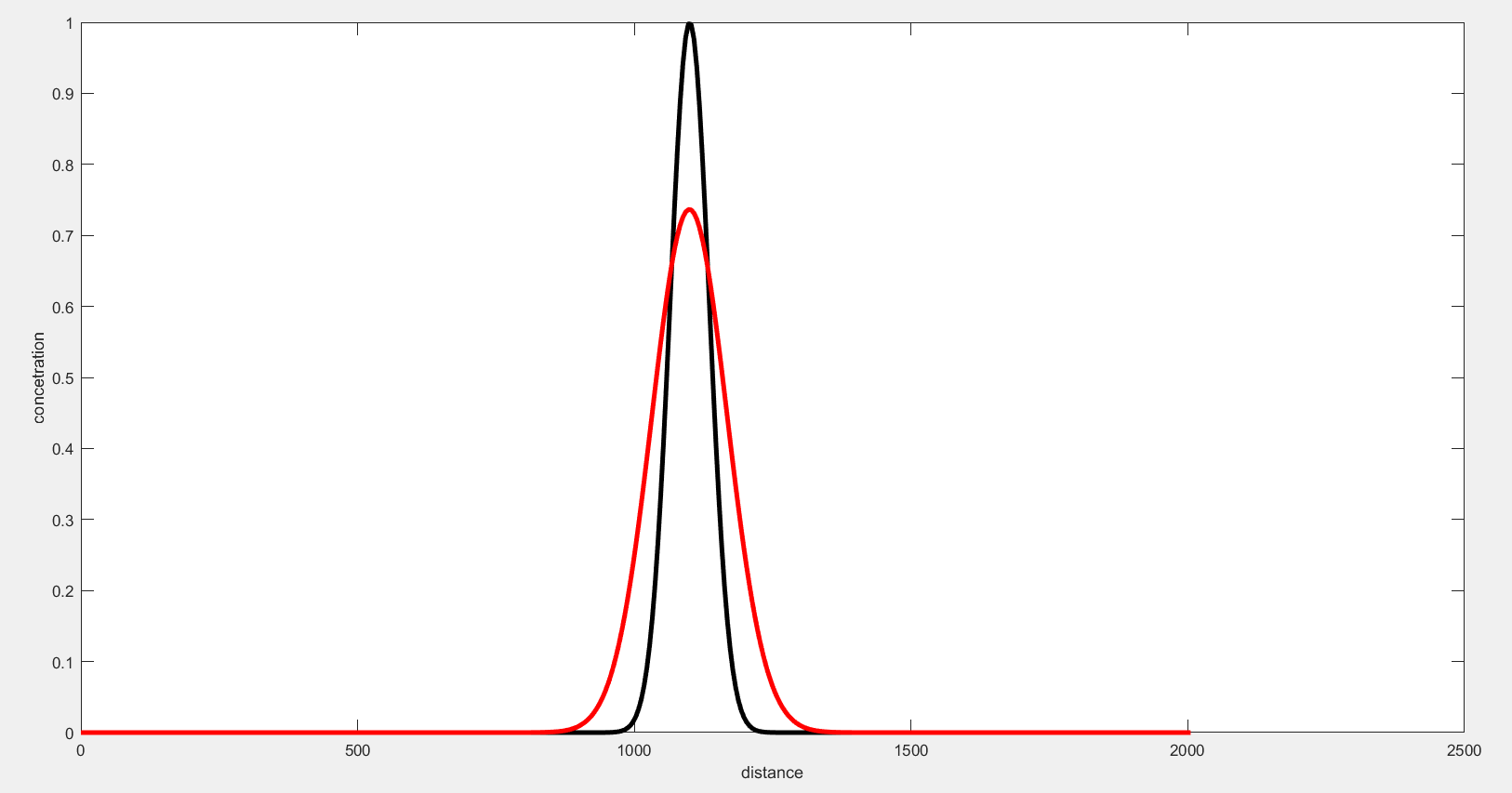MATLAB代码改进版，对图片进行了一点修饰。
% This is a  First Order Upwind for Advection Equation
clear
clc

% Set the parameters
xmin = 0;
xmax = 2000;
N = 400;
dx = (xmax-xmin)/N;
tmin = 0;
tmax = 1000;
dt = 3;
u = 1;
sigma = 110;
x0 = 600;

% Set the initial condition
x = linspace(xmin, xmax+dx, N+1);
C0 = exp( -(x-x0).^2/sigma^2*0.5);
C0(1) = 0; %MATLAB Array index start from 1

Cplus = C0;
nstep = tmax/dt;

for n = 1:nstep

Ctemp  = Cplus;
% calculate the new value
for i = 2:N+1
Cplus(i) = Ctemp(i) - u*dt*(Ctemp(i) - Ctemp(i-1))/dx;
end

end

% calculate exact solution
Cexact = exp( -(x-x0-u*tmax).^2/sigma^2*0.5);

% visualization
figure(1)
L = plot(x,Cexact,'k-',x,Cplus,'r-','linewidth',1.2);
legend(L,'解析解','\sigma0=110','location','NorthEast','FontName','宋体');
xlabel('\itX \rm(m)');
ylabel('\itC \rm');
axis([1100 2100 0 1]);
set(gca,'FontName','Times New Roman','Fontsize',10.5);
set(get(gca,'xlabel'),'FontName','Times New Roman','Fontsize',10.5);
set(get(gca,'ylabel'),'FontName','Times New Roman','Fontsize',10.5);
grid on;
set(gcf,'PaperUnits','centimeter','PaperPosition',[0 0 16 9]);

为什么想到用Matlab?是因为我用Python绘制时不知道如何绘制解析解的图像，真是个青菜啊。
Matlab报错信息1:矩阵求幂的维度不正确
解决办法：请检查并确保矩阵为方阵并且幂为标量。要执行按元素矩阵求幂，请使用 【.^】。比较搞笑的是，系统都提示我了，我竟然还花了一定时间才找到问题所在。
一维常系数对流方程（Python编写思路主要来源）
展开全文python
• matlab中，利用迎风离散格式求解对流方程的代码。 给定初值和边界条件，直接求出数值解。
• 主要需要的专业知识：计算热物理，matlab编程语言（研究生课程，还是需要一些基础知识的） 举个栗子： 对于具有常扩散系数的一维扩散方程： 其中D是常数，初始条件为： 边界条件为： 按照将方程和定解条件无量纲化，...
主要需要的专业知识：计算热物理，matlab编程语言（研究生课程，还是需要一些基础知识的）
举个栗子：
对于具有常扩散系数的一维扩散方程：

其中D是常数，初始条件为：

边界条件为：

按照将方程和定解条件无量纲化，并且在无量纲化条件下进行求解。
解：
无量纲化之后的结果为（我这里就默认大家都会无量纲化了哈）：

其中w为无量纲化后的物理量，T为无量纲时间，X为无量纲化距离。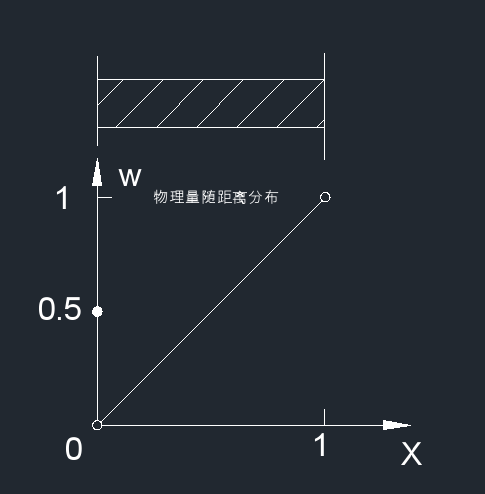下面进入编程阶段：
%%copyright...explict FTCS

clear;
clc;
tic;
n=0;
t=1000;
T=0.002;%T=0.001收敛%T=0.002发散
L=0.05;
e=1;

//固定坐标轴
axis([0,1,0,1]);
axix=0:0.05:1;
//物理量w的取值范围为0到1，题目要求起始和结束都为0.5
w=0:0.05:1;
w(1)=0.5;
w(length(w))=0.5;
w1=w;
//利用动态作图drawnow功能
h = plot(axix,w,'YDataSource','w');
photo = figure(1);
xlim([0 1]);ylim([0 1]);hold on;

//FTCS算法核心，挺简单的
for time=1:t
while max(e)>0.0001
n=n+1;
for x=2:length(w)-1;
w1(x)=w(x)+T/L/L.*(w(x+1)-2.*w(x)+w(x-1));
end
e=abs(w1-w)./w;
w=w1;
fprintf('caculation times=%d\n',n);
refreshdata(h, 'caller');
drawnow
pause(0.5)
end
break;
end
//tictoc显式运行时间
toc;

运行出来的图片如下：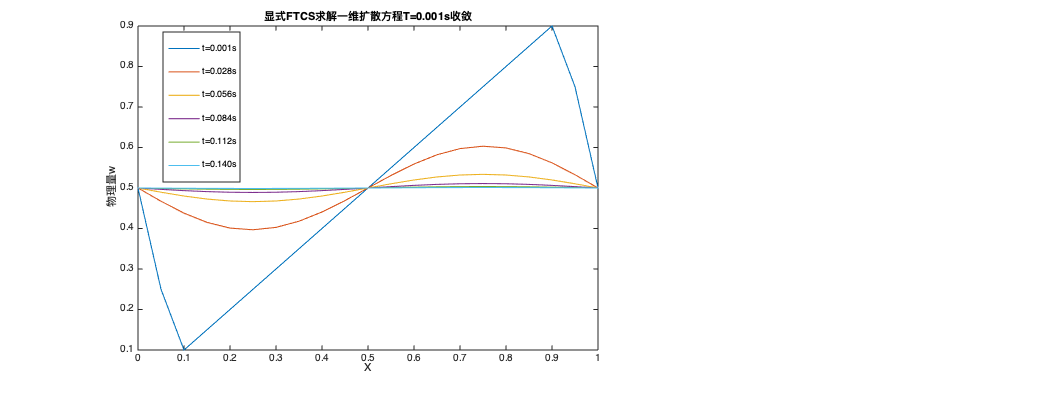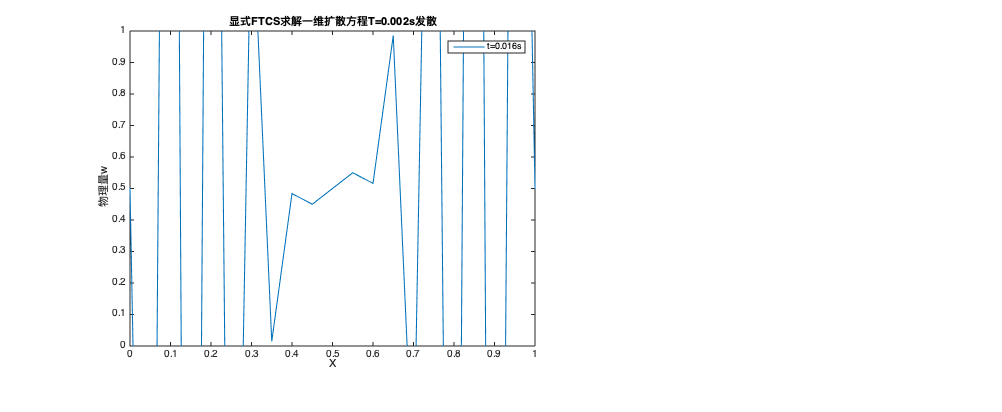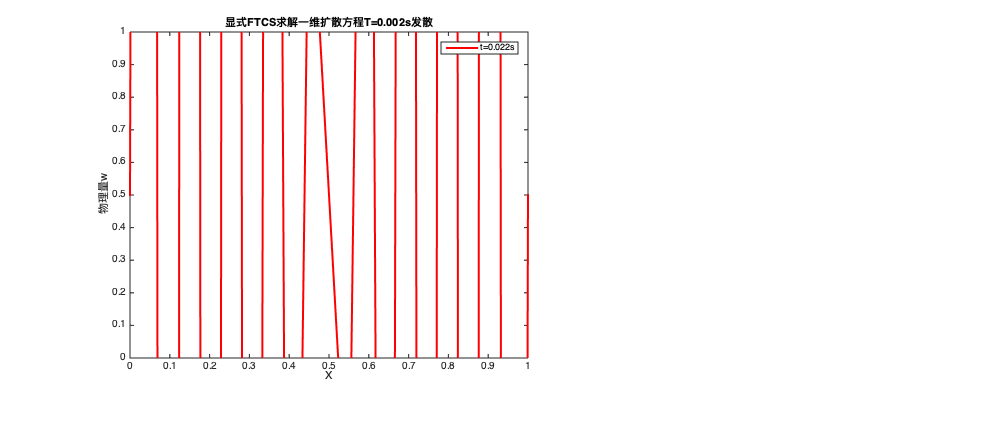分析：
稳定是要达到一定条件的，对于此题可以需要满足；时不稳定，时数值模拟的结果是正确的，并且在0.112s时基本达到稳定状态。


展开全文算法
• 一维非稳态扩散算例一样的初始和边界条件 ，采用乘方格式 时间步长为0.001s，初始温度场为200，速度为2m/s，长度为2cm，t=0s时刻东侧温度突然降至0C。时间差分采用全隐式格式
• 1. 一维常系数对流方程的步长定律和固有差分格式 The Step Law and Natural Difference Scheme for the One-dimensional Advection Equation with Constant Coefficients 2. 一维常系数对流方程的步长定律和固有差...PDE数值解
• 2、第次接触matplotlib中ion()和ioff()。ion()开启了交互模式,ioff() 感觉像暂停图像 同时，查阅其他博客得到： python可视化库matplotlib有两种显示模式： 阻塞（block）模式 交互（interactive）模式 在...
• 使用各种有限差分方案（Lax-Friedrichs，Lax-Wendroff，MacCormack和具有四阶显式人工黏度项的隐式Beam-Warming）以保守形式模拟非线性对流方程。 所使用的初始条件是Heaviside阶跃函数，并且预计该波将向右传播（平...
• 这项工作基于具有对流和源/消耗项的一维稳态传热方程。 如上所述，这种方法非常简单，但是我们在这里尝试开发一种代码，学生可以轻松使用该代码来开发或理解本课程的概念。 如果您发现有趣的东西，请不要忘记“拉取...
• 物理模型： 有对流换热的细杆导热，一端固定，恒温边界，一端自由，绝热边界。python
• 对于具有均匀初始等离子体浓度的一维气体二极管，该程序使用均匀细网格上的线法（MOL）求解连续性的电子和离子方程对流（漂移）通量用 Lax-Friedrichs 表达式分割，并用五阶加权 ENO 方案 (WENO5-LF) 重建。 扩散...
• 个简单的有限体积工具该代码是化学/石油工程师开发种简单的工具来求解对流扩散方程的一般形式的结果： α∂ϕ /∂t+∇。（uϕ）+∇。（-D∇ϕ）+ βϕ =γ 在1D，1D轴对称（径向），2D，2D轴对称（圆柱）和3​​...
• 对于一维对流方程，列出了三种常见差分格式（FTFS、FTBS、FTCS）的求解过程，利用matlab进行求解并得出结果，可以发现，FTFS和FTBS差分格式均可以计算出一维对流方程的数值解，而FTCS差分格式计算过程发散，数值格式...
• 对于一维对流方程（如下），这里列出了三种常见的差分格式求解。 利用MATLAB进行求解 1、FTFS格式 2、FTBS格式 3、FTCS格式 综上，FTFS和FTBS差分格式均可以计算出一维对流方程的数值解，而FTCS差分格式计算...差分方程...

# 一维对流扩散方程matlabmatlab 订阅